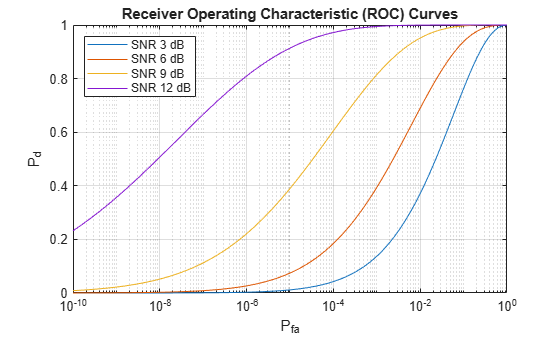# rocsnr

Receiver operating characteristic curves by SNR

## Syntax

``````[Pd,Pfa] = rocsnr(SNRdB)``````
``````[Pd,Pfa] = rocsnr(SNRdB,Name=Value)``````
``rocsnr(___)``

## Description

``````[Pd,Pfa] = rocsnr(SNRdB)``` returns the single-pulse detection probabilities, `Pd`, and false-alarm probabilities, `Pfa`, for the SNRs in the vector `SNRdB`. By default, for each SNR, the detection probabilities are computed for 101 false-alarm probabilities between `1e–10` and `1`. The false-alarm probabilities are logarithmically equally spaced. The ROC curve is constructed assuming a coherent receiver with a nonfluctuating target.```

example

``````[Pd,Pfa] = rocsnr(SNRdB,Name=Value)``` returns detection probabilities and false-alarm probabilities with additional options specified by one or more name-value arguments.```
``rocsnr(___)` plots the ROC curves.`

## Examples

collapse all

Plot ROC curves for different SNRs for a single pulse.

```SNRdB = [3 6 9 12]; [Pd,Pfa] = rocsnr(SNRdB,SignalType="NonfluctuatingCoherent"); semilogx(Pfa,Pd) grid on xlabel("P_{fa}") ylabel("P_d") legend("SNR "+SNRdB+" dB",Location="northwest") title("Receiver Operating Characteristic (ROC) Curves")```## Input Arguments

collapse all

Signal-to-noise ratios in decibels, specified as a row or column vector.

Example: `[3 6 9 12]`

Data Types: `double`

### Name-Value Arguments

Specify optional pairs of arguments as `Name1=Value1,...,NameN=ValueN`, where `Name` is the argument name and `Value` is the corresponding value. Name-value arguments must appear after other arguments, but the order of the pairs does not matter.

Example: `MinPfa=1e-8,NumPoints=64,NumPulses=10`

Before R2021a, use commas to separate each name and value, and enclose `Name` in quotes.

Example: `'MinPfa',1e-8,'NumPoints',64,'NumPulses',10`

Maximum false-alarm probability to include in the ROC calculation, specified as a positive scalar.

Data Types: `double`

Minimum false-alarm probability to include in the ROC calculation, specified as a positive scalar.

Data Types: `double`

Number of pulses to integrate when calculating the ROC curves, specified as a positive integer. A value of `1` indicates no pulse integration.

Data Types: `double`

Number of SNR values to use when calculating the ROC curves, specified as a positive integer. The actual values are equally spaced between `MinSNR` and `MaxSNR`.

Data Types: `double`

This property specifies the type of received signal or, equivalently, the probability density functions (PDF) used to compute the ROC. Valid values are: `"Real"`, `"NonfluctuatingCoherent"`, `"NonfluctuatingNoncoherent"`, `"Swerling1"`, `"Swerling2"`, `"Swerling3"`, and `"Swerling4"`. Values are not case sensitive.

The `"NonfluctuatingCoherent"` signal type assumes that the noise in the received signal is a complex-valued, Gaussian random variable. This variable has independent zero-mean real and imaginary parts each with variance σ2/2 under the null hypothesis. In the case of a single pulse in a coherent receiver with complex white Gaussian noise, the probability of detection, PD, for a given false-alarm probability, PFA is:

`${P}_{D}=\frac{1}{2}\text{erfc}\left({\text{erfc}}^{-1}\left(2{P}_{FA}\right)-\sqrt{\chi }\right)$`

where `erfc` and `erfc-1` are the complementary error function and that function’s inverse, and χ is the SNR not expressed in decibels.

For details about the other supported signal types, see .

Data Types: `char` | `string`

## Output Arguments

collapse all

Detection probabilities corresponding to the false-alarm probabilities, returned as a vector. For each SNR in `SNRdB`, `Pd` contains one column of detection probabilities.

False-alarm probabilities, returned as a column vector. By default, the false-alarm probabilities are 101 logarithmically equally spaced values between `1e–10` and `1`. To change the range of probabilities, use the optional `MinPfa` or `MaxPfa` input argument. To change the number of probabilities, use the optional `NumPoints` input argument.

## Version History

Introduced in R2011a